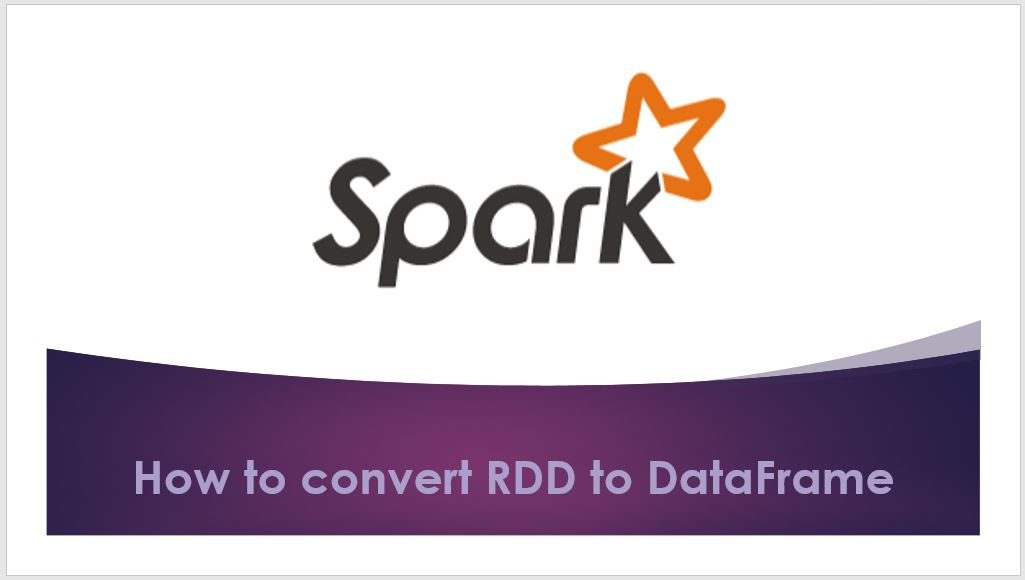# Convert Spark RDD to DataFrame | Dataset

While working in Apache Spark with Scala, we often need to Convert Spark RDD to DataFrame and Dataset as these provide more advantages over RDD. For instance, DataFrame is a distributed collection of data organized into named columns similar to Database tables and provides optimization and performance improvement.

In this article, I will explain how to Convert Spark RDD to Dataframe and Dataset using several examples.

## Create Spark RDD

First, let’s create an RDD by passing Seq object to `sparkContext.parallelize()` function. We would need this “rdd” object for all our examples below.

``````
import spark.implicits._
val columns = Seq("language","users_count")
val data = Seq(("Java", "20000"), ("Python", "100000"), ("Scala", "3000"))
val rdd = spark.sparkContext.parallelize(data)
``````

## Convert Spark RDD to DataFrame

Converting Spark RDD to DataFrame can be done using toDF(), createDataFrame() and transforming `rdd[Row]` to the data frame.

### Convert RDD to DataFrame – Using toDF()

Spark provides an implicit function `toDF()` which would be used to convert RDD, Seq[T], List[T] to DataFrame. In order to use toDF() function, we should import implicits first using `import spark.implicits._`.

``````
val dfFromRDD1 = rdd.toDF()
dfFromRDD1.printSchema()
``````

By default, toDF() function creates column names as “_1” and “_2” like Tuples. Outputs below schema.

``````
root
|-- _1: string (nullable = true)
|-- _2: string (nullable = true)
``````

`toDF() `has another signature that takes arguments to define column names as shown below.

``````
val dfFromRDD1 = rdd.toDF("language","users_count")
dfFromRDD1.printSchema()
``````

Outputs below schema.

``````
root
|-- language: string (nullable = true)
|-- users_count: string (nullable = true)
``````

By default, the datatype of these columns infers to the type of data and set’s nullable to true. We can change this behavior by supplying schema using StructType – where we can specify a column name, data type and nullable for each field/column.

### Convert RDD to DataFrame – Using createDataFrame()

`SparkSession `class provides `createDataFrame()` method to create DataFrame and it takes rdd object as an argument. and chain it with toDF() to specify names to the columns.

``````
val columns = Seq("language","users_count")
val dfFromRDD2 = spark.createDataFrame(rdd).toDF(columns:_*)``````

Here, we are using scala operator `<strong>:_*</strong>` to explode columns array to comma-separated values.

### Using RDD Row type RDD[Row] to DataFrame

Spark `createDataFrame()` has another signature which takes the RDD[Row] type and schema for column names as arguments. To use this first, we need to convert our “rdd” object from RDD[T] to RDD[Row]. To define a schema, we use StructType that takes an array of StructField. And StructField takes column name, data type and nullable/not as arguments.

``````
//From RDD (USING createDataFrame and Adding schema using StructType)
val schema = StructType(columns
.map(fieldName => StructField(fieldName, StringType, nullable = true)))
//convert RDD[T] to RDD[Row]
val rowRDD = rdd.map(attributes => Row(attributes._1, attributes._2))
val dfFromRDD3 = spark.createDataFrame(rowRDD,schema)
``````

This creates a data frame from RDD and assigns column names using schema.

## Convert Spark RDD to Dataset

The DataFrame API is radically different from the RDD API because it is an API for building a relational query plan that Spark’s Catalyst optimizer can then execute.

The Dataset API aims to provide the best of both worlds: the familiar object-oriented programming style and compile-time type-safety of the RDD API but with the performance benefits of the Catalyst query optimizer. Datasets also use the same efficient off-heap storage mechanism as the DataFrame API.

DataFrame is an alias to Dataset[Row]. As we mentioned before, Datasets are optimized for typed engineering tasks, for which you want types checking and object-oriented programming interface, while DataFrames are faster for interactive analytics and close to SQL style.

About data serializing. The Dataset API has the concept of encoders which translate between JVM representations (objects) and Spark’s internal binary format. Spark has built-in encoders that are very advanced in that they generate byte code to interact with off-heap data and provide on-demand access to individual attributes without having to de-serialize an entire object.

``````
val ds = spark.createDataset(rdd)
``````

## Conclusion:

In this article, you have learned how to convert Spark RDD to DataFrame and Dataset, we would need these frequently while working in Spark as these provides optimization and performance over RDD.

Happy Learning !!

### NNK

SparkByExamples.com is a Big Data and Spark examples community page, all examples are simple and easy to understand and well tested in our development environment Read more ..

### This Post Has 4 Comments

1.Anonymous

Really Great tutorial with scala .i have cleared my interview by following this tutorial.
it would be great if you can make this tutorial as a PDF ,so that people can use this as a reference .

2.PANKAJ

Very Good Article. Look forward to seeing such awesome articles with examples/explanations

1.NNK

Thanks Pankaj.

3.Anonymous

Nice Article. Keep posting good articles